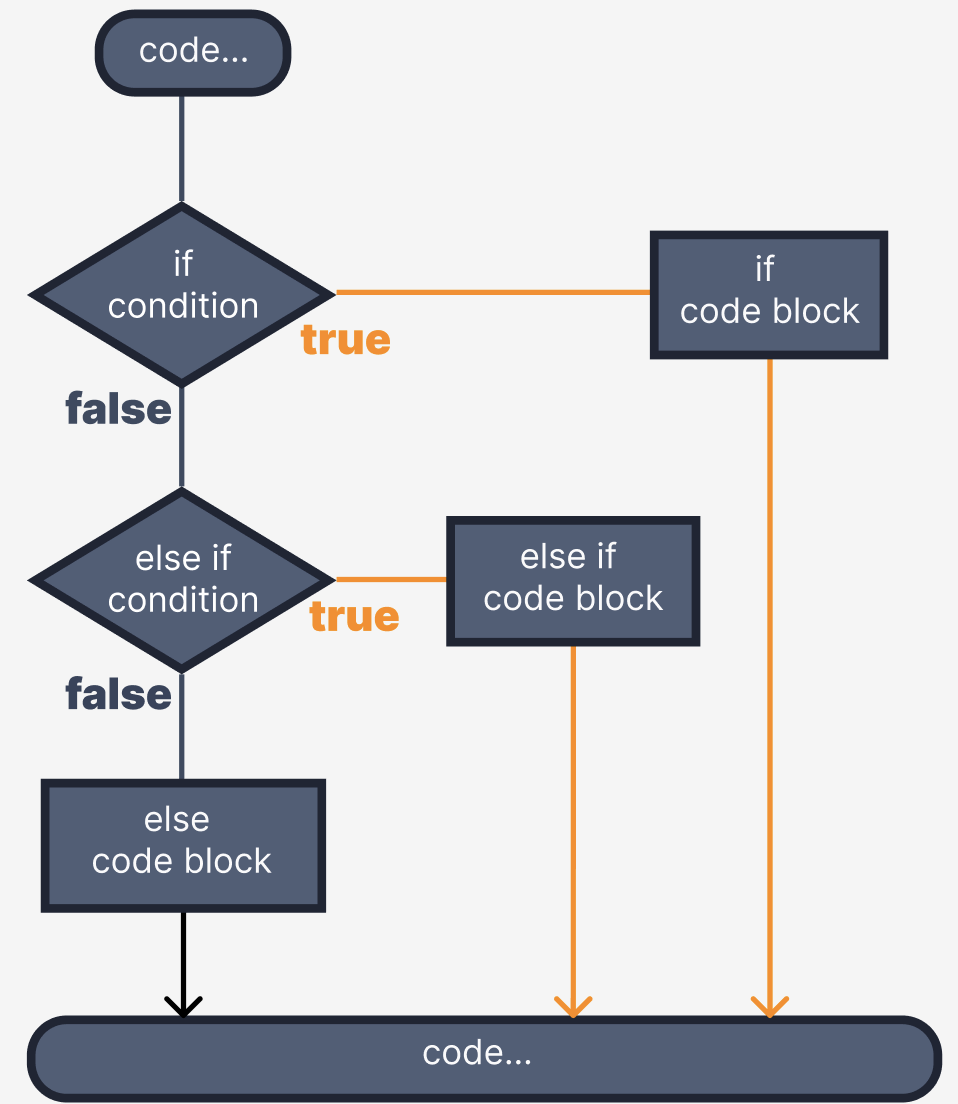Course Content

# Introduction to Dart

1. First Acquaintance with Dart

2. Variables and data types

3. Conditional statements

4. List and String

Introduction to Dart

# else if statement

The `else…if` statement is useful to test multiple conditions. Following is the syntax of the same.• An `if` construct can contain any number of `else if` blocks of code.
• It is not necessary to use the code block `else`.

### Syntax

The `else if` syntax is like the `if` syntax:

`else if` keyword, value in the parentheses `()` and a code block in the curly brackets `{}`.

If one of the conditions is met, then after executing its code block, the subsequent conditions will not be checked, and the code blocks of those conditions will not be executed either.

### Examplemain.dartIn this program, we have a variable `num` which is equal to `2`. We use a conditional statement if to check if num is greater than `0`. If this condition is `true` (meaning `num` is a positive number), it prints the message `"is positive"`. Otherwise, if `num` is less than `0`, it prints `"is negative"`. If none of these conditions is `true` (i.e., num is equal to `0`), it prints `"is zero"`.

Fix the code so that the program works correctly.

else if (score is double)
``````

``````main.dartEverything was clear?

Section 3. Chapter 3